Next: Scenarios for SUSY Breaking Up: SUPERSTRING PHENOMENOLOGY AND THE Previous: Gaugino Condensation

# Soft SUSY Breaking Terms

It was found many years ago that some terms can be added to a supersymmetric lagrangian that do not respect supersymmetry but still keep the soft ultraviolet behaviour of the theory. These are the soft-breaking terms. They are naturally generated after supersymmetry breaking in general supergravity models and correspond to the following terms

(i) Scalar masses, implying that the scalars such as squarks, become usually heavier than the fermions of the same multiplet. These are terms in the Lagrangian of the form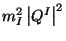.

(ii) Gaugino masses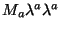splitting the gauge multiplets.

(iii)Cubic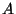terms. Cubic scalar terms in the potential related to Yukawa couplings and controlled by arbitrary dimensionfull coefficients () of order the gravitino mass.

(iv)The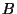-term. A quadratic term in the potential for the scalars of the form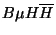where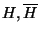represent the Higgs fields and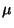is a constant that gives rise to a term in the original superpotential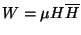which is allowed by all the symmetries of the minimal supersymmetric standard model. Sinceis a dimensionfull parameter it causes a problem to introduce it in the supersymmetric Lagrangian since it has to be of the order of the gravitino mass and there is no reason that a term in the supersymmetric lagrangian knows the scale of the breaking of supersymmetry.This is known as theproblem and several solutions have been proposed. Depending on the proposed solution there is an expression for the parameterafter supersymmetry breaking. In particular, if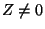in eq. (21), it can be seen that theterm is generated after supersymmetry breaking. Some calculations have shown that there are models for which(for recent discussions see [45,95] ).

In this section we will follow the following strategy. Treat the supersymmetry breaking mechanism as a black box, but based on the experience with gaugino condensation, use that the end result of this mechanism is to induce nonvanishing values to the auxiliary fields of the moduli or dilaton fields. Therefore we can parametrize our ignorance of the particular breaking mechanism by working with general values of these auxiliary fields. Let us for simplicity treat a single modulus field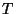and the dilaton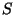. But the analysis has been done in more general cases [96,60,97,98]. We can then write the goldstino field (Goldstone fermion eaten by the gravitino in the process of supersymmetry breaking) as a linear combination of the fermionic components ofand: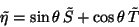(44)

where the goldstino angle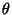mixing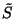and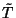describes the relative contribution ofandto the breaking of supersymmetry. The general procedure for extracting the soft breaking terms is clear. We start with the supersymmetric lagrangian and substitute in it the nonvanishing auxiliary fields (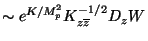), using expression (44). Performing the so called flat space limit in which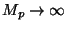with fixed gravitino mass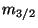(representing the nonvanishing vev's of the auxiliary fields and parametrizing the breaking of supersymmetry. We end up with the following values for the soft breaking parameters :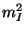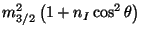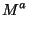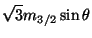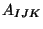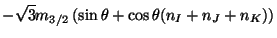(45)

From this we can extract several conclusions. The dilaton dominated scenario for which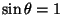, the soft breaking parameters are universal! This is a very appealing result explaining one of the less justified assumptions of the minimal supersymmetric standard model. On the other hand, this scenario is so restrictive that it is relatively easy to rule it out. Something that was claimed recently after comparison with the value of the top quark mass, using a particular solution of theproblem above (see for instance  for a recent discussion). The importance of this scenario is that eqs (45) forare valid in general and not only for orbifold models. For arbitrary mixing angle, the soft breaking terms are not necessarily universal (unless special values of the modular weights are taken). In that case we have to confront problems with flavour changing neutral currents . Another conclusion we can extract from the form of the soft breaking terms is that for negative modular weights, we can get tachyons in the spectrum, for certain values of the mixing angle. The same condition that avoids tachyons, implies that the gaugino masses have to be bigger than the scalar masses, unless both vanish; in that case loop corrections may be important to determine the relative masses.

SubsectionsNext: Scenarios for SUSY Breaking Up: SUPERSTRING PHENOMENOLOGY AND THE Previous: Gaugino Condensation
root 2001-01-22